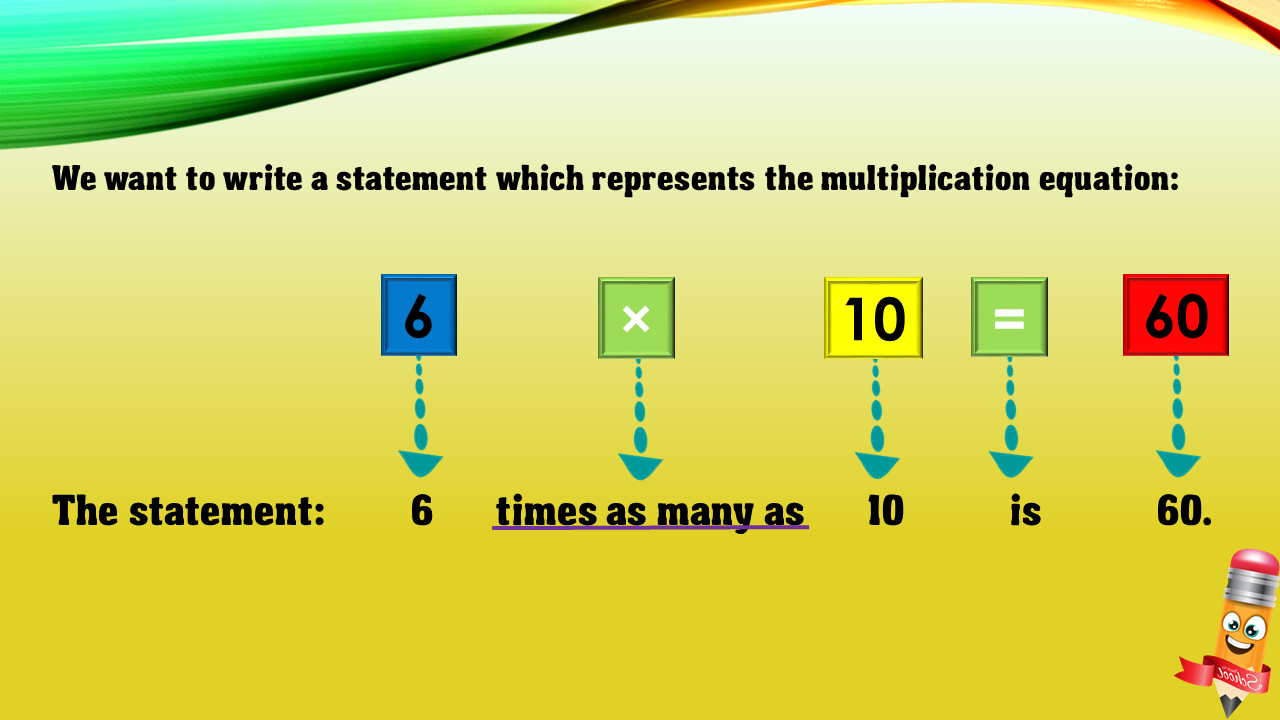1
visibility

Choose one statement which represents the equation:
6 × 10 = 60 ?

6 times as many as 10 is 60.
6 more than 10 is 60.
10 less than 60 is 6.
10 times as many as 60 is 6.

• A

6 times as many as 10 is 60.

• B

6 more than 10 is 60.

• C

10 less than 60 is 6.

• D

10 times as many as 60 is 6.